# ElectrochemistryPage 1

#### WATCH ALL SLIDES

Slide 1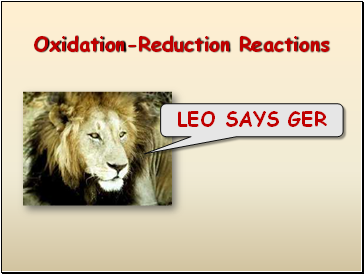Oxidation-Reduction Reactions

LEO SAYS GER

Slide 2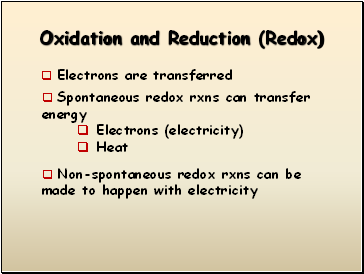## Oxidation and Reduction (Redox)

Electrons are transferred

Spontaneous redox rxns can transfer energy

Electrons (electricity)

Heat

Non-spontaneous redox rxns can be made to happen with electricity

Slide 3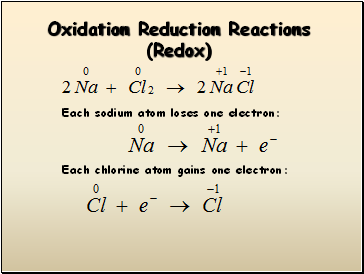## Oxidation Reduction Reactions (Redox)

Each sodium atom loses one electron:

Each chlorine atom gains one electron:

Slide 4## LEO says GER :

Lose Electrons = Oxidation

Sodium is oxidized

Gain Electrons = Reduction

Chlorine is reduced

Slide 5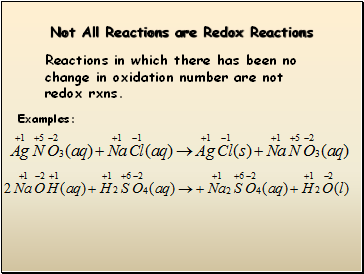## Not All Reactions are Redox Reactions

Reactions in which there has been no change in oxidation number are not redox rxns.

Examples:

Slide 6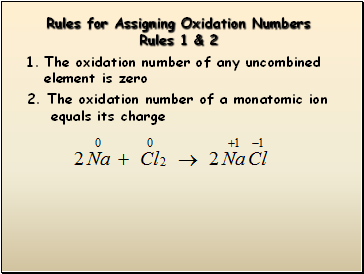## Rules for Assigning Oxidation Numbers

Rules 1 & 2

The oxidation number of any uncombined element is zero

2. The oxidation number of a monatomic ion

equals its charge

Slide 7Rules for Assigning Oxidation Numbers Rules 3 & 4

3. The oxidation number of oxygen in

compounds is -2

4. The oxidation number of hydrogen in compounds is +1

Slide 8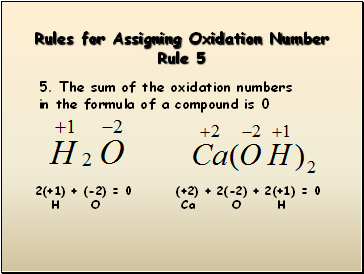Rules for Assigning Oxidation Number Rule 5

5. The sum of the oxidation numbers in the formula of a compound is 0

2(+1) + (-2) = 0

H O

(+2) + 2(-2) + 2(+1) = 0

Ca O H

Slide 9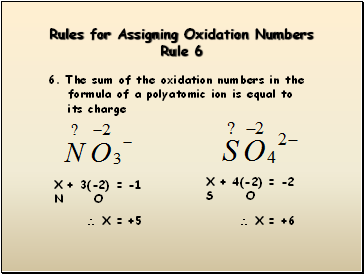Rules for Assigning Oxidation Numbers Rule 6

6. The sum of the oxidation numbers in the

formula of a polyatomic ion is equal to

its charge

X + 3(-2) = -1

N O

 X = +5

 X = +6

X + 4(-2) = -2

S O

Slide 10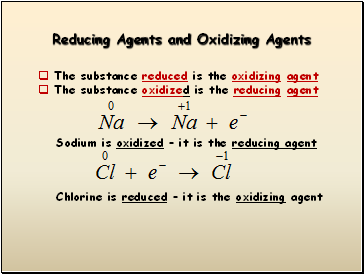## Reducing Agents and Oxidizing Agents

The substance reduced is the oxidizing agent

The substance oxidized is the reducing agent

Sodium is oxidized – it is the reducing agent

Chlorine is reduced – it is the oxidizing agent

Slide 11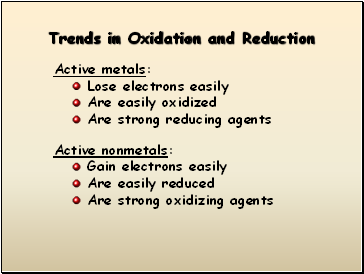## Trends in Oxidation and Reduction

Active metals:

Lose electrons easily

Are easily oxidized

Are strong reducing agents

Go to page:
1  2